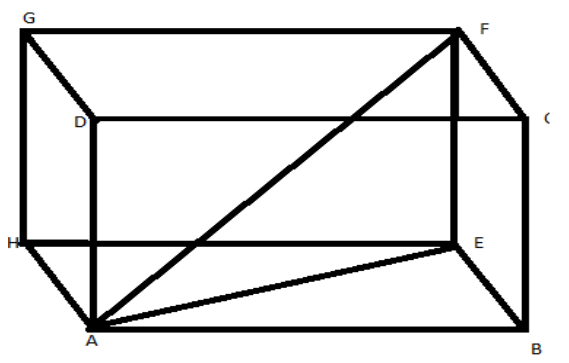QUESTION

# The length, breadth and height of a room are 8m 50cm, 6m 25cm and 4m 75cm respectively. Find the length of the longest rod that can measure the dimensions of the room exactly.

Hint: In order to solve this problem you should know that the longest rod for a cuboid is equal to the length of the body diagonal of the cuboid.Given,
Length(AB) = 8m 50cm = 8.5m
Breadth(EB) = 6m 25cm = 6.25m
Height(EF) = 4m 75cm = 4.75m
To find the length of body diagonal, we first need to find the length of a face diagonal AE and then find the body diagonal AF.
(using Pythagoras’s theorem)
In $\Delta$AEB,
$A{E^2} = A{B^2} + B{E^2}$
$A{E^2} = {(8.5)^2} + {(6.25)^2} \\ AE = \sqrt {72.25 + 39.06} \\ AE = \sqrt {111.31} \\ AE = 10.5m \\ \\$
Now to find the face diagonal AF
In $\Delta$AEF,
$A{F^2} = A{E^2} + F{E^2} \\ A{F^2} = {(10.5)^2} + {(4.75)^2} \\ AF = \sqrt {111.31 + 22.56} \\ AF = \sqrt {133.87} \\ AF = 11.57m \\$
Hence, the length of the longest rod for measurement is 11.57m.

Note: To solve such problems we must know the concept of longest rod and application of Pythagoras Theorem to find the body diagonal of the cuboid, as similarly the body diagonal of a cube can be found. Proceeding like this it will solve your problem.